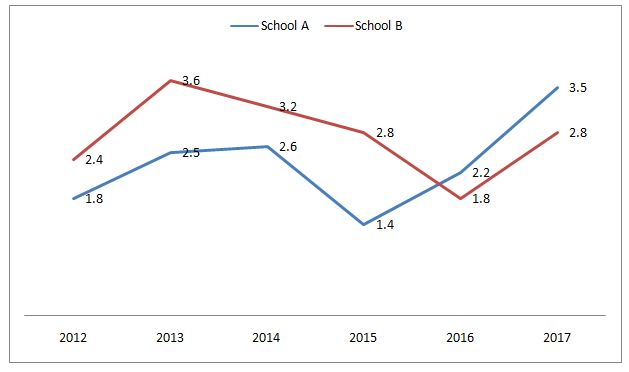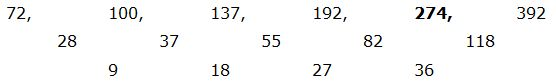# NIACL AO Mains– Quantitative Aptitude Questions Day- 11

Dear Readers, Bank Exam Race for the Year 2019 is already started, To enrich your preparation here we have providing new series of Practice Questions on Quantitative Aptitude – Section. Candidates those who are preparing for NIACL AO Mains 2019 Exams can practice these questions daily and make your preparation effective.

[WpProQuiz 5164]

Directions (Q. 1 – 5): Find the wrong term in the following number series

1) 23, 24.1, 26.3, 29.6, 34, 39.6

a) 39.6

b) 24.1

c) 26.3

d) 29.6

e) 34

2) 72, 100, 137, 192, 273, 392

a) 100

b) 137

c) 273

d) 192

e) 392

3) 258, 256, 265, 237, 300, 176

a) 256

b) 176

c) 237

d) 300

e) 265

4) 4, 7, 20, 78, 394, 2363

a) 20

b) 78

c) 394

d) 2363

e) 4

5) 3, 11, 49, 210, 969, 5061

a) 11

b) 969

c) 210

d) 5061

e) 49

Directions (Q. 6 – 10): Study the following information carefully and answer the given questions.

The following line graph shows the ratio of no of boys to the number of girls passed from two different schools in the year 2012 to 2017.6) If in the year 2014, the total number of boys in School A is 78 and the total number of girls in School B in the year 2013 is 80, then find the difference between the total number of girls in School A in the year 2014 to that of boys in School B in the year 2013?

a) 212

b) 306

c) 258

d) 344

e) None of these

7) The number of boys passed in School A in the year 2015 is approximately what percentage of the number of girls passed in School A in the same year?

a) 125 %

b) 140 %

c) 112 %

d) 105 %

e) None of these

8) If the number of girls passed from School A in the year 2016 and the no of girls passed from School B in the year 2017 is equal, then the number of boys passed from school A in the year 2016 is what approximately percentage more/less than the number of boys passed from School B in the year 2017?

a) 21 % less

b) 27 % more

c) 27 % less

d) 21 % more

e) 35% more

9) If the number of boys passed from School A in the year 2012 is 108, which is equal to the number of boys passed from School B in the year 2013, then find the ratio of number of girls passed from School A in the year 2012 to that of total number of girls passed from School A in the year 2012 and School B in the year 2013?

a) 8: 5

b) 2: 3

c) 7: 4

d) 4: 1

e) None of these

10) In which year, the highest number of girls passed in School A?

a) 2013

b) 2015

c) 2016

d) Can’t be determined

e) None of these

Directions (1-5):

The correct series is,

23, 24.1, 26.3, 29.6, 34, 39.5

The pattern is, +1.1, +2.2, +3.3, +4.4, +5.5,…

The wrong term is, 39.6

The correct series is,The difference of difference is, 9, 18, 27, 36,…

The wrong term is, 273

The correct series is,

258, 256, 265, 237, 302, 176

The pattern is, -(13 +1), +(23 +1), -(33 +1), +(43+1), -(53 +1),…

The wrong term is, 300

The correct series is,

4, 7, 20, 79, 394, 2363

The pattern is, *2 – 1, *3 – 1, *4 – 1, *5 – 1, *6 – 1,….

The wrong term is, 78

The correct series is,

3, 11, 49, 211, 969, 5061

The pattern is, *1 + 23, *2 + 33, *3 + 43, *4 + 53,….

The wrong term is, 210

Directions (6-10):

In the year 2014, the ratio of boys to girls in School A = 2.6 = 26/10 = 13/5

The total number of boys in School A is 78

= > 13’s = 78

= > 1’s = 6

Total girls in School A = 5’s = 30

In the year 2013, the ratio of boys to girls in School B = 3.6 = 36/10 = 18/5

The total number of girls in School B = 80

= > 5’s = 80

= > 1’s = 16

Total number of boys in School B = 18*16 = 288

Required difference = 288 – 30 = 258

The ratio of boys to that of girls passed in School A in 2015 = 1.4

= > 14/10 = 7/5

Required % = (7/5)*100 = 140 %

Let the number of girls passed from School A in the year 2016 and the number of girls passed from School B in the year 2017 be x,

The ratio of boys to girls in School A in 2016,

= > (BA/GA) = 2.2

= > BA = 2.2x

The ratio of boys to girls in School B in 2017,

= > (BB/GB) = 2.8

= > BB = 2.8x

Required % = [(2.8x – 2.2x)/2.8x]*100 = (0.6/2.8)*100

= 21.42 % = 21 % less

The ratio of number of boys to that of girls in School A in 2012

= > 1.8 = 18/10 = 9/5

The number of boys passed from School A in the year 2012 = 108

= > 9’s = 108

= > 1’s = 12

The number of girls passed from School A in the year 2012 is,

= > 5’s = 5*12 = 60

The ratio of number of boys to that of girls in School B in 2013

= > 3.6 = 36/10 = 18/5

The number of boys passed from School B in the year 2013 = 108

= > 18’s = 108

= > 1’s = 6

The number of girls passed from School B in the year 2013 is,

= > 5’s = 30

Required ratio = 60: 90 = 2: 3